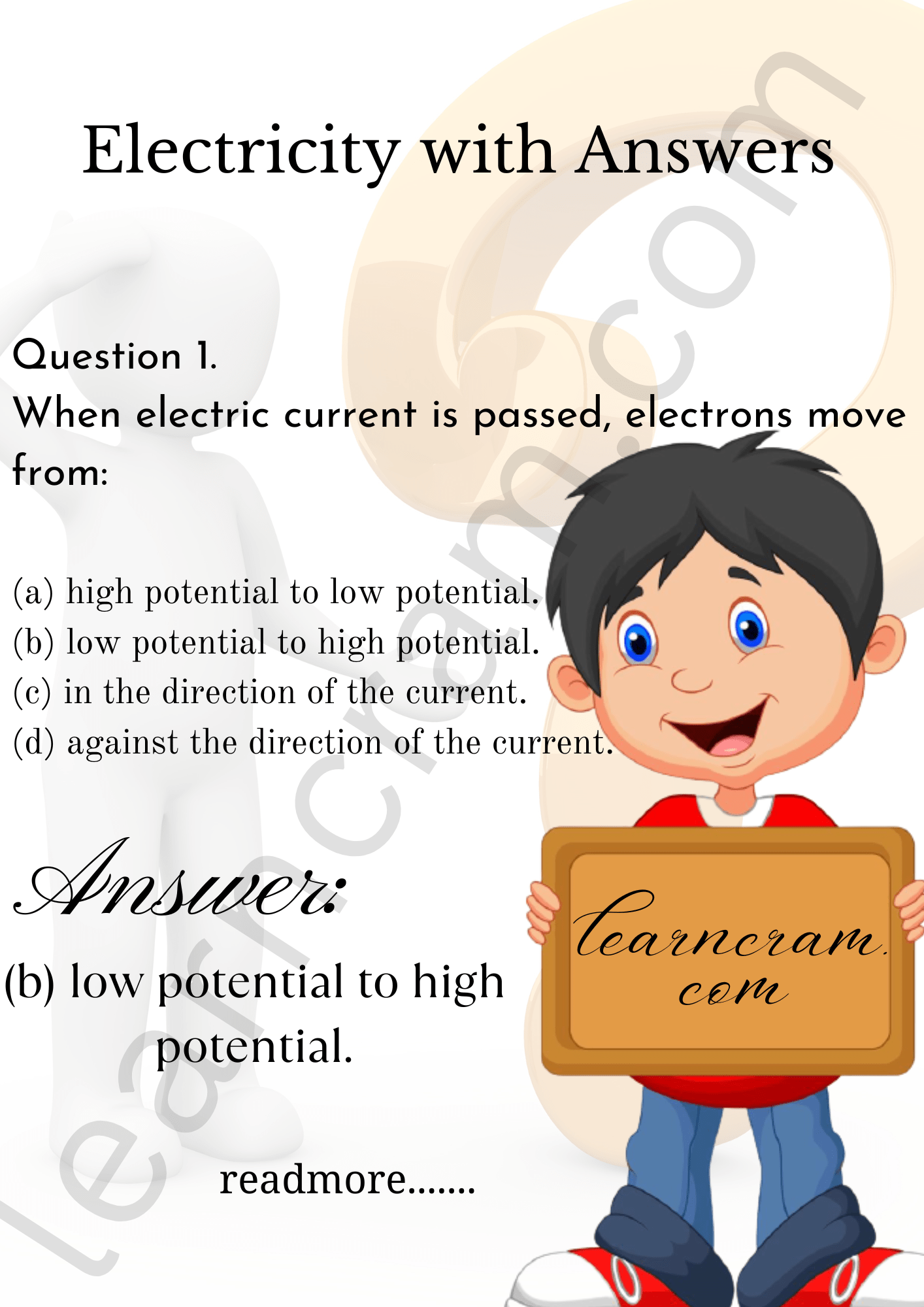# MCQ Questions for Class 10 Science Chapter 12 Electricity with Answers

We have compiled the NCERT MCQ Questions for Class 10 Science Chapter 12 Electricity with Answers Pdf free download covering the entire syllabus. Practice MCQ Questions for Class 10 Science with Answers on a daily basis and score well in exams. Refer to the Electricity Class 10 MCQs Questions with Answers here along with a detailed explanation.

## Electricity Class 10 MCQs Questions with Answers

1. When electric current is passed, electrons move from:
(a) high potential to low potential.
(b) low potential to high potential.
(c) in the direction of the current.
(d) against the direction of the current.2. The heating element of an electric iron is made up of:
(a) copper
(b) nichrome
(c) aluminium
(d) iron3. The electrical resistance of insulators is
(a) high
(b) low
(c) zero
(d) infinitely high

4. Electrical resistivity of any given metallic wire depends upon
(a) its thickness
(b) its shape
(c) nature of the material
(d) its length

Electricity Class 10 MCQ Pdf Question 5. Which of the following is not correctly matched?6. Electric power is inversely proportional to
(a) resistance
(b) voltage
(c) current
(d) temperature

MCQ Chapter Electricity Class 10 Question 7. What is the commercial unit of electrical energy?
(a) Joules
(b) Kilojoules
(c) Kilowatt-hour
(d) Watt-hour

8. Three resistors of 1 Ω, 2 ft and 3 Ω are connected in parallel. The combined resistance of the three resistors should be
(a) greater than 3 Ω
(b) less than 1 Ω
(c) equal to 2 Ω
(d) between 1 Ω and 3 Ω

Explaination:9. An electric bulb is connected to a 220V generator. The current is 0.50 A. What is the power of the bulb?
(a) 440 W
(b) 110 W
(c) 55 W
(d) 0.0023 W

Explaination:
Here, V = 220 V, I = 0.50 A
∴ Power (P) = VI = 220 x 0.50 = 110 W

10. The resistivity of insulators is of the order of
(a) 10-8-m
(b) 101-m
(c) 10-6-m
(d) 106-m

11. 1 kWh = ……….. J
(a) 3.6 × 10-6 J
(b) $$\frac{1}{3.6}$$ × 106 J
(c) 3.6 × 106 J
(d) $$\frac{1}{3.6}$$ × 10-6 J

12. Which of the following gases are filled in electric bulbs?
(a) Helium and Neon
(b) Neon and Argon
(c) Argon and Hydrogen
(d) Argon and Nitrogen

13. 100 J of heat is produced each second in a 4Ω resistor. The potential difference across the resistor will be:
(a) 30 V
(b) 10 V
(c) 20 V
(d) 25 V

14. Electric potential is a:
(a) scalar quantity
(b) vector quantity
(c) neither scalar nor vector
(d) sometimes scalar and sometimes vector

Electricity Question 15. 1 mV is equal to:
(a) 10 volt
(b) 1000 volt
(c) 10-3 volt
(d) 10-6 volt

Electricity MCQ Question 16. Coulomb is the SI unit of:
(a) charge
(b) current
(c) potential difference
(d) resistance

Fill in the Blanks

1. The SI unit of current is ……… .
2. According to ……… Law, the potential difference across the ends of a resistor is directly proportional to the ……… through it, provided its remains constant.
3. The resistance of a conductor depends directly on its ……… , inversely on its ……… and also on the ……… of the conductor.
4. The SI unit of resistivity is ……… .
5. If the potential difference across the ends of a conductor is doubled, the current flowing through it, gets ……… .

1. ampere
2. Ohm’s, current, temperature
3. length, area of cross-section, material
4. ohm-metre (Ω m)
5. doubled

Hope the information shed above regarding NCERT MCQ Questions for Class 10 Science Chapter 12 Electricity with Answers Pdf free download has been useful to an extent. If you have any other queries of CBSE Class 10 Science Electricity MCQs Multiple Choice Questions with Answers, feel free to reach us so that we can revert back to us at the earliest possible.

### 2 thoughts on “MCQ Questions for Class 10 Science Chapter 12 Electricity with Answers”

1.It was very helpful for me … it helped me to understand the chapter in a better way …the whole chapter was covered in this questions ..Now I think that I am fully prepared for the test …thanks allot

2.Mastery app thanks